# Lesson 13: The hydrogen atom

13.1 Energy levels of the hydrogen atom by means of box-shaped potential approximation – 13.2 Determination of the potential well width – 13.3 Determination of the potential well depth
13.4 Determination of the energy values – 13.5 Progress check – 13.6 Summary

In this lesson, a standard problem of quantum physics is considered: The hydrogen atom. You will learn about a method which can be used to calculate the energy levels approximately with tools available in schools. The Coulomb potential of the nucleus is replaced here by a suitably adapted box-shaped potential.¹ This method has the advantage that all approximations have already been carried out in the classical potential. The subsequent quantum mechanical calculation is then conducted without any approximations.

### 13.1 Energy values of the hydrogen atom by means of box-shaped potential approximation

With the hydrogen atom, a single electron is present in the Coulomb potential of the atomic nucleus. It cannot possess arbitrary values of the total energy; its energy is quantized. The discussion of the energy quantization in the hydrogen atom is a key aspect of the atomic physics lesson.

The   quantum mechanical calculation of the energy levels of the hydrogen atom cannot be carried out in schools. We are restricted to simplified models and approximations. The most frequently used method is to go back to Bohr’s model of the atom ( overview of the approaches to the hydrogen atom used in school textbooks).

However: If we say the objective of the quantum physics lesson is to turn our backs on classical ways of thinking, then using Bohr’s model of the atom is problematic, because the electrons circle on well-defined orbits here, as they do according to classical physics. We have to fall back on concepts which no longer have a justification in quantum mechanics (positions and paths of electrons), which foster classical, incorrect ideas in the minds of the pupils.

The Coulomb potential of the atomic nucleus is replaced by a “similar” potential: By a box-shaped potential with infinitely high potential walls. More precisely, it is a three-dimensional, box-shaped potential well with width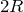and the energy difference “W_0” between the “bottom” of the potential well and the zero point of the energy. We have to find values for the width and the energy difference so that the potential well approximates the Coulomb potential as well as possible.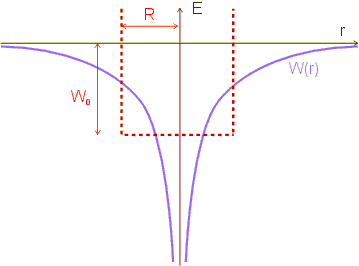### 13.2 Determination of the potential well width

In classical physics, a bound electron (energy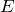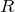) cannot penetrate into the wall, because its potential energy would otherwise be greater than its total energy.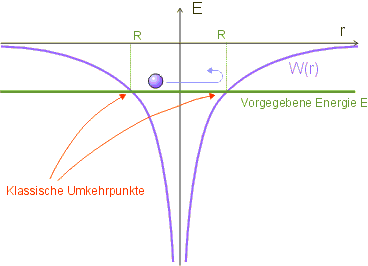The turning point is therefore characterized by the fact that the kinetic energy is zero; the total energyis then equal to the potential energy: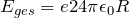or solving for: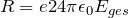The maximum distanceis therefore a function of the energy.

We have thus obtained a classical estimate for the region in which the electron is to be found. The Coulomb potential is replaced by a potential well of widthin our model; within this region, the potential is assumed to be constant, the region outside is not accessible to the electron (infinitely high potential walls).

### 13.3 Determination of the potential well depth

If we want to solve this problem graphically and without the mathematics still required for the slightly more exact averaging of the Coulomb potential over a sphere with radius, we take the following route: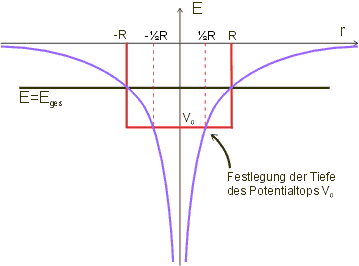As we can see from the graph, for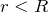the potential is always below the horizontal line at the total energy. The value of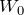must therefore be below Etot. We obtain a simple estimate by specifying thatshould have the value of the Coulomb potential at the position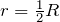(graph). The following therefore applies (inserting the expression for(cf. above)):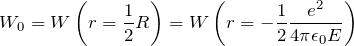With the explicit expression for the Coulomb potential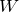, this gives: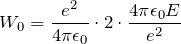and the final result foris: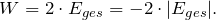Potential problem in understanding when matching the zero point of the energy.

### 13.4 Determination of the energy values

The classical model potential, which provides a good approximation for the Coulomb potential, is specified by the results obtained so far. The parameters of the three-dimensional, infinite potential well were determined to be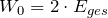and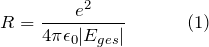.
We can now continue with the quantum mechanical calculation of the energy values.
The energy levels for the three-dimensional potential well are already known: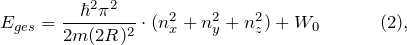with the electron mass m and the quantum numbers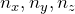. We limit ourselves to states which do not distinguish a specific spatial direction. Then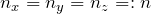applies so that the electron state is described by only a single quantum number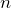. The energy levels from Equation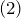then become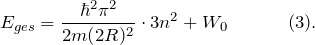If we insert the parametersanddetermined above, we obtain: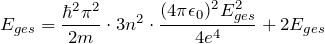and from this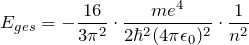This is the final result of our model calculation for the hydrogen atom. If we compare this to the exact result, we find that the energy levels are correct apart from the factor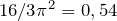.

¹ W. R. Theis, Begründung diskreter Eigenwerte für gebundene Zustände ohne Lösung der Eigenwertgleichung, Physik und Didaktik 22 (3), 198 (1994)

### 13.5 Progress check

The following points were important in this chapter:

• Simplification of the hydrogen problem by adapting a potential well with infinitely high walls
• Energy eigenvalues of the hydrogen potential.

Before you move on to the next chapter, make sure you know the fundamental ideas behind these points. You can then check this with the aid of the Summary.

### 13.6 Summary of Chapter 13: The hydrogen atom

In schools, the Schrödinger equation for the hydrogen atom cannot be solved exactly. This chapter therefore presents an approximation method which can be used to obtain the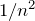dependence of the energy eigenvalues.

The fundamental idea consists in approximating the Coulomb potential by a suitably selected potential well with infinitely high walls. The classical turning points of the motion determine the width of the potential well.

A self-consistent equation to determine the energy eigenvalues is obtained.

The method can also be used for other potentials. The condition is that the corresponding classical motion is similarly limited by the turning points.